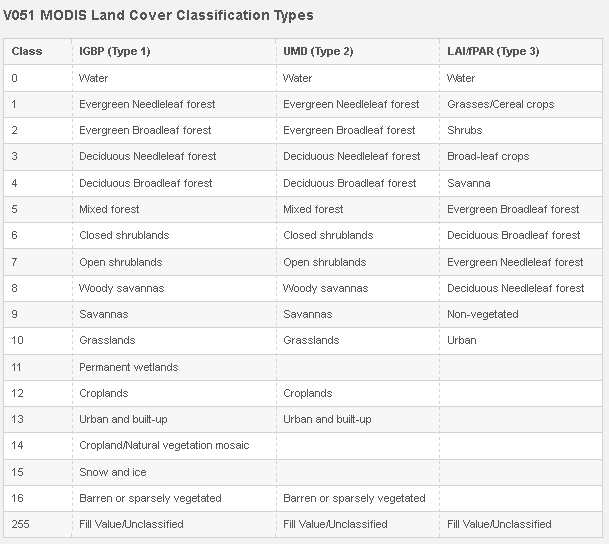### Ecosystem modeling: a look up table for land cover and vegetation types

Vegetation type is an important factor in ecosystem modeling. In the 3D ecosystem modeling, the MODIS land cover product is used. Both the IGBP and UMD schemes are used in separate modules.### Spatial datasets operations: mask raster using region of interest

Climate change related studies usually involve spatial datasets extraction from a larger domain.
In this article, I will briefly discuss some potential issues and solutions.

In the most common scenario, we need to extract a raster file using a polygon based shapefile. And I will focus as an example.

In a typical desktop application such as ArcMap or ENVI, this is usually done with a tool called clip or extract using mask or ROI.

Before any analysis can be done, it is the best practice to project all datasets into the same projection.

If you are lucky enough, you may find that the polygon you will use actually matches up with the raster grid perfectly. But it rarely happens unless you created the shapefile using "fishnet" or other approaches.

What if luck is not with you? The algorithm within these tool usually will make the best estimate of the value based on the location. The nearest re-sample, but not limited to, will be used to calculate the value. But what about the outp…

### Numerical simulation: ode/pde solver and spin-up

For Earth Science model development, I inevitably have to deal with ODE and PDE equations. I also have come across some discussion related to this topic, i.e.,

https://www.researchgate.net/post/What_does_one_mean_by_Model_Spin_Up_Time

In an attempt to answer this question, as well as redefine the problem I am dealing with, I decided to organize some materials to illustrate our current state on this topic.

Models are essentially equations. In Earth Science, these equations are usually ODE or PDE. So I want to discuss this from a mathematical perspective.

Ideally, we want to solve these ODE/PDE with initial condition (IC) and boundary condition (BC) using various numerical methods.
https://en.wikipedia.org/wiki/Initial_value_problem
https://en.wikipedia.org/wiki/Boundary_value_problem

Because of the nature of geology, everything is similar to its neighbors. So we can construct a system of equations which may have multiple equation for each single grid cell. Now we have an array of equation…

### Watershed Delineation On A Hexagonal Mesh Grid: Part A

One of our recent publications is "Watershed Delineation On A Hexagonal Mesh Grid" published on Environmental Modeling and Software (link).
Here I want to provide some behind the scene details of this study.

(The figures are high resolution, you might need to zoom in to view.)

First, I'd like to introduce the motivation of this work. Many of us including me have done lots of watershed/catchment hydrology modeling. For example, one of my recent publications is a three-dimensional carbon-water cycle modeling work (link), which uses lots of watershed hydrology algorithms.
In principle, watershed hydrology should be applied to large spatial domain, even global scale. But why no one is doing it?  I will use the popular USDA SWAT model as an example. Why no one is setting up a SWAT model globally?
There are several reasons we cannot use SWAT at global scale: We cannot produce a global DEM with a desired map projection. SWAT model relies on stream network, which depends on DEM.…